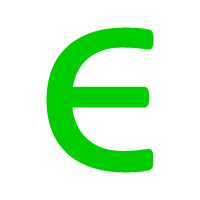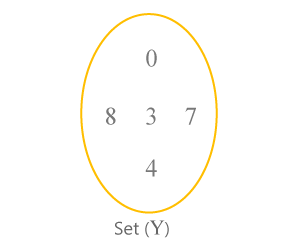Belongs toA symbol, used to give the meaning “Belongs to” in mathematics is defined as the symbol of Belongs to.

The term “Belongs to” is often used in mathematics especially in set theory. It is essential to use it in mathematical form expressions too but it cannot be used as it is, in mathematical language. So, a Latin based symbol ($\in$) was introduced in mathematics to use in place of “Belongs to” in mathematical language.

ExampleConsider a set $Y$ and assume elements of this set are $0,3,4,7$ and $8$. It is written in set form in mathematics as given here.

$Y=\left\{0,3,4,7,8\right\}$

So, the elements $0,3,4,7$ and $8$ belong to set $Y$. Therefore, each element of this set can be written in mathematical language.

• The element ‘$0$‘ belongs to Set $Y$. It is written in mathematics as $0\in Y$
• The element ‘$3$‘ belongs to Set $Y$. It is written in mathematics as $3\in Y$
• The element ‘$4$‘ belongs to Set $Y$. It is written in mathematics as $4\in Y$
• The element ‘$7$‘ belongs to Set $Y$. It is written in mathematics as $7\in Y$
• The element ‘$8$‘ belongs to Set $Y$. It is written in mathematics as $8\in Y$

As demonstrated here, the symbol belongs to $\left(\in \right)$ is used in mathematics.

Latest Math Topics
Latest Math Problems
Email subscription
Math Doubts is a best place to learn mathematics and from basics to advanced scientific level for students, teachers and researchers. Know more